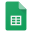# Enumerative Combinatorics mini Workshop 2012

The Enumerative Combinatorics mini Workshop 2012 (ECmW2012) will be held at KAIST on Feburary 21-22. If you want to participate, please write a registration form at the bottom of this page and email to ecmw2102@combinatorics.kr until Feburary 14.

The purpose of this workshop is not just presenting individual works but sharing problems together. So the topic is limited to "enumeration" of combinatorial objects, and the style of talk tends to be less formal.## Information

• Title Enumerative Combinatorics mini Workshop 2012

• Date Feb. 21-22 (Tue-Wed), 2012

• Venue Room #1409, Building E6-1, KAIST, Daejeon

• We are going to

• give just 5 talks without contributed talks.

• provide for 3 meals (lunch & dinner of 21, lunch of 22) of all participants.

• support the accommodation of graduate students who registered until Feb 14.

## Talks

• Talk I

• Speaker 서승현 (강원대학교)

• Language Korean for speaking, English for writing

• Title Refined enumeration of trees by the size of maximal decreasing trees

• Abstract Let ${\mathcal T}_{n}$ be the set of rooted labeled trees on $\set{0,...,n}$. Given a rooted labeled tree $T$, A maximal decreasing subtree of a rooted labeled tree is defined by the maximal subtree from the root with all edges being decreasing. Recently Seo and Shin have studied a new refinement ${\mathcal T}_{n,k}$ of ${\mathcal T}_n$, which is the set of rooted labeled trees whose maximal decreasing subtree has $k+1$ vertices. In this talk, we introduce the refinement of the set of labeled ordered trees by the size of maximal decreasing trees. We also present a refinement of the set of labeled $p$-ary trees. This is a joint work with Heesung Shin.

• Talk II

• Speaker 유환철 (KIAS)

• Language

• Title Specht modules of general diagrams and their Hecke counterparts

• Abstract There are natural representations, called Specht modules, of the permutation group $\S_n$ parameterized by diagrams, or equivalently by bicolored graphs. How they decompose into irreducibles is often related to many interesting enumeration problems. In the first half of this talk, Specht modules for some of the well-studied families of diagrams are going to be discussed. Their dimensions and decomposition rules are explained. In the second half of the talk, the approach using Hecke algebras is going to be discussed.

• Talk III

• Speaker 신희성 (인하대학교)

• Language Korean

• Title q-Hermite 다항식을 포함하는 두 항등식에 관하여

• Abstract 이 발표에서는 q-Hermite 다항식을 포함하고 있는 두 항등식을 제시한다. 이 항등식들의 양변의 계수가 정수임을 확인하고, 이 계수들을 설명할 수 있는 조합론적인 대상을 만들어 본다. 그리고 이 대상에 일대일 대응을 주어 이 항등식이 증명할 수 있는지 확인해 보고, 토론해 보려고 한다. 하나의 항등식은 김동수 교수님과 다른 하나의 항등식은 Jiang Zeng 교수님과의 공동 연구 결과이다.

• Talk IV

• Speaker 조수진 (아주대학교)

• Language

• Title Skew Schur P-functions

• Abstract There are two combinatorial models for Schur P-functions; marked shifted tableaux and semistandard decomposition tableaux. Skew marked shifted tableaux can be defined in a natural way to define skew Schur P-functions, yet a natural definition of skew semistandard decomposition tableaux is not known.

Starting with basic combinatorial models and properties of (skew) Schur P-functions, the problem of defining skew semistandard decomposition tableaux will be setup while the falsity of the conjecture by L. Serrano is explained.

A problem on Littlewood-Richardson coefficients for Schur P-functions also will be introduced if time permitted.

• Talk V

• Speaker 김상욱 (전남대학교)

• Language

• Title Flag vectors of polytopes

• Abstract The flag vector of a polytope contains all enumerative incidence information between the faces of the polytope. Although all linear equations for the flag vector of polytopes are given by the generalized Dehn-Sommerville equations, linear inequalities are not characterized yet. In this talk, we introduce the cd-index which compactly encodes the flag vectors of polytopes and give recent results on linear inequalities for flag vectors using cd-index.

## Discussion session

• Problem I

• Speaker Fu, Shishuo (KAIST)

• Abstract Characterization and enumeration of k-shape partition.

• Problem II

• Speaker 주형관 (전남대학교)

• Abstract For a simple graph G=(V,E) with |V|=k , we define a polytope P=P(G) such that P={(x_1,x_2, \cdots, x_k ) \in R^k | x_i \ge 0, x_i1 + x_i2 \le 1 iff v_i1 is adjacent to v_i2 in G}. Can we find any relation(s) between the specrum of the adjacency matrix A(G) of the graph G and the coefficients of the Ehrhart polynomial of the polytope P(G)?ECmW2012 - 기존 사이트 도구의 목록 페이지